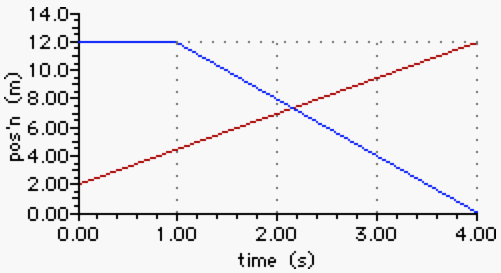# Mechanics: 1-Dimensional Kinematics

## 1-D Kinematics: Audio Guided Solutions

#### Problem 12:

The position-time graph below represents the motion of two students - Mac (in red) and Tosh (in blue) - as they enter and exit the school library during a passing period.Use the graph to determine the speeds at which the two students move. (Ignore any stationary periods of time.) Then determine how much faster the fastest student moves than the slower student.

## Audio Guided Solution

Click to show or hide the answer!

## Habits of an Effective Problem Solver

• Read the problem carefully and develop a mental picture of the physical situation. If necessary, sketch a simple diagram of the physical situation to help you visualize it.
• Identify the known and unknown quantities in an organized manner. Equate given values to the symbols used to represent the corresponding quantity - e.g., vo = 0 m/s; a = 4.2 m/s/s; vf = 22.9 m/s; d = ???.
• Use physics formulas and conceptual reasoning to plot a strategy for solving for the unknown quantity.
• Identify the appropriate formula(s) to use.
• Perform substitutions and algebraic manipulations in order to solve for the unknown quantity.

Get more information on the topic of Kinematics at The Physics Classroom Tutorial.